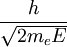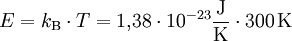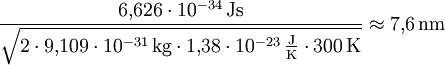# What is the quantum dot

A Quantum dot (engl. quantum dot) is a nanoscopic material structure, mostly made of semiconductor material (e.g. InGaAs, CdSe or GaInP / InP). Charge carriers (electrons, holes) in a quantum dot are so limited in their mobility in all three spatial directions that their energy can no longer assume continuous, but only discrete values. Quantum dots behave similarly to atoms, but their shape, size or the number of electrons in them can be influenced. This enables the electronic and optical properties of quantum dots to be tailored. Typically, their own atomic order of magnitude is around 104 Atoms. If it is possible to arrange several individual quantum dots in close proximity to each other so that charge carriers (especially electrons) can hop from one to the next quantum dot via tunneling processes, one speaks of quantum dot molecules.

### Methods of making quantum dots

• Wet chemical methods (e.g. cadmium selenide, ZnO): the actual quantum dot is surrounded by additional layers to improve the optical properties, water solubility or biocompatibility.
• Molecular beam epitaxy: self-organized quantum dots are formed from thin layers (a few nanometers or < 5="" atomlagen)="" an="" grenzflächen="" zwischen="" verschiedenen="" halbleiterschichten,="" zum="" beispiel="" durch="" die="" volmer-weber-="" oder="" die="" stranski-krastanov-methode.="" die="" ursache="" für="" die="" selbstorganisation="" findet="" sich="" in="" der="" durch="" die="" verschiedenen="" gitterkonstanten="" der="" beiden="" materialien="" (substrat="" und="" quantenpunkt)="" entstehenden="" verspannung="" der="" quantenpunktschicht.="" die="" ecs-theorie="">equilibrium crystal shape - equilibrium crystal form) of thermodynamics makes the prediction that a macroscopic inclusion with a fixed volume in thermodynamic equilibrium takes the form that minimizes the surface free energy. This leads to small elevations (islands) forming from the quantum dot layer above a certain layer thickness. This process also reduces the tension within the islands - another driving force behind agglomeration.
• Lithography: the quantum dot is 'written' on a substrate by means of electron beams, AFM or the like and then 'exposed' by a suitable etching process (wet / dry etching). The resulting mesas can now be left free-standing or - to improve the electronic or optical properties - be enclosed again by a suitable semiconductor material by 'growing' a further layer. During the structuring process, the quantum dot can also be provided with electrical leads. The disadvantage of this method is the accumulation of lattice defects caused by the etching, which leads to deteriorated electronic and thus also optical properties of the quantum dot.

### Magnitude

The size of the quantum dot is in the range of the De Broglie wavelength of the electron, because this is where the quantum properties come to light. De Broglie wavelength for an electron at room temperature:The following applies here:It results:For holes, the higher mass with these quantum dot sizes results in a weaker 'confinement', which means that the line-like energy structure is not as pronounced.

### spectrum

Due to the previously determined size of the quantum dot, atom-like states are formed. The transition from the classic ribbon model of semiconductor physics to the quantized energy levels of low-dimensional solids is continuous and depends on the strength of the confinement of the wave function of the charge carrier located in the quantum dot or, more precisely, its wave function.

The spectrum of a quantum dot is now defined by the energy emitted when the charge carriers recombine. As expected, this should be a line spectrum for atom-like quantized states. Now the dipole oscillation, which leads to a spectral line, has to be understood as a damped harmonic oscillator with finite damping. With the Fourier transformation of the envelope (spectral analysis does nothing else) from spatial space into the frequency domain, a Lorentz curve is obtained, the width of which depends on the attenuation constant. It is said that the spectral lines are 'Lorentz broadened'.

A quantum dot ensemble, i.e. several quantum dots, have a Gaussian curve as a common spectrum. This reflects the Gaussian size distribution of the quantum dots around a statistically frequent value that was favored by the growth process.